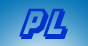Interesting Math Problem Math Problems Home  |  Home  |  Send Feedback

There are 50 pennies lying on a table, 10 heads up, 40 tails up.
Your task is to divide the pennies into two piles
such that each pile has the same number of heads.

The piles do not need to have the same number of coins.
You may flip pennies as you divide them.
You are blindfolded, and have no way to distinguish heads from tails.

Divide the coins into a pile of 40 and a pile of 10 at random.

The heads will be divided somehow between the two piles.
N in one, and 10 - N in the other, with 0 <= N <= 10.

The original coin distribution is then
 10-N 10-N Pile 1 Pile 2 Total: 10 40 Heads: N 10-N Tails: 10-N 30+N Flip all 10 coins in the Pile 1, and you will have Heads: Tails: N 30+N

It doesn't matter what N is.